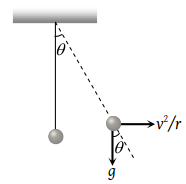A car is moving in a circular horizontal track of radius 10 m with a constant speed of 10 m/sec. A plumb bob is suspended from the roof of the car by a light rigid rod of length 1.00 m. The angle made by the rod with track is

(1) Zero

(2) 30°

(3) 45°

(4) 60°

Concept Videos :-

#24-Intro-to-Uniform-Circular-Motion
#25-Centripetal-Acceleration
#26-Centripetal &Tangential-Acceleration
#27-Solved-Examples-12
#28-Solved-Examples-13
#29-Solved-Examples-14
#30-Solved-Examples-15
#31-Solved-Examples-16
#32-Kinematics -Uniform-Circular-Motion
#33-Solved-Examples-17

Concept Questions :-

Circular motion

(3) $\mathrm{tan}\theta =\frac{{v}^{2}\text{​}/\text{​}r}{g}=\frac{{v}^{2}}{rg}$$\theta ={\mathrm{tan}}^{-1}\left(\frac{{v}^{2}}{rg}\right)={\mathrm{tan}}^{-1}\left(\frac{10×10}{10×10}\right)$

$\theta ={\mathrm{tan}}^{-1}\left(1\right)=45°$

Difficulty Level:

• 18%
• 10%
• 70%
• 4%